# The Fifteen Puzzle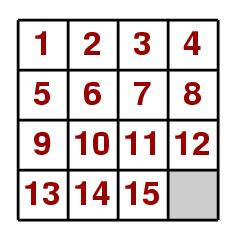The Fifteen Puzzle has been around for over a hundred years, and has been a craze for almost every generation. The basic form is of a 4 by 4 grid usually made with sliding tiles in a tray. There are 15 tiles numbered 1 to 15 and the 16th place is empty as shown. Before play begins the tiles are randomly scrambled by sliding to any starting position. The object of the game is then to unscramble the tiles (by sliding, not lifting them) to get them into consecutive order, with the space in the bottom right again. (This will be referred to as the home position.)

Sam Loyd was the man who invented the 14-15 or Boss puzzle. This was a version where the starting position was very similar to the home position except that the 14 and 15 were inverted. In the early 1870's he offered a \$1000 reward to anyone who could solve it, which set off "fifteen fever". However no-one claimed the prize for the simple reason that it is not solvable! The reason shall dealt with later.

### Permutations, Interchanges And Parity

It can be seen that the puzzle has 15 tiles and a space distributed over 16 squares. The number of different arrangements of tiles can be calculated: the first square has 16 possible numbers/space to choose from, the 2nd will have 15 possibilities...etc so there are 16 factorial possible arrangements. This is over 20 billion different arrangements. It shall be seen however that only 16!/2 of these are solvable.

To understand the principles involved, first look at a simpler case of permutations (or arrangements) of a 2 by 2 grid, with 3 tiles; 1,2,3.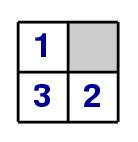These are 3 of the arrangements of the grid, with the first picture being the home state; 9 other arrangements can be found by cycling the 1 round to a new position and then rearranging the 2 and 3. Of course there are 4! = 24 possible arrangements, but the other 12 can not be reached by simple sliding moves. They would be found by performing an interchange; ie where one tile is moved to the position of another tile or the space, which in turn is moved somewhere else, possibly to the first tile's original position.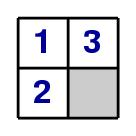(ie swapping two of the tiles). One can see that the following arrangement is not possible from the home state by following legal moves (ie sliding not lifting):

Cycles can be used to describe the permutations of objects through interchanges. Lifting a tile up is not a legal operation, but a cycle of interchanges may describe a set of legal sliding moves in a shorter form. For example (3 1 2 *) would be the cycle fromto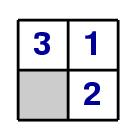, where * is the empty space. Of course one can see that this is the same as (2 * 3 1) . In a similar way, (2 * 1) would be the permutation ofto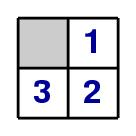. As the 3 is not mentioned, there is an implication that it is left in its original position. Thus the impossible arrangement seen previously would have a cycle from the home state of the form (2 3) , which can easily been seen to be an interchange that is not a legal permutation. It shall be seen later how to tell generally if a particular permutation is legal for a particular starting state.

Cycles can be strung together to get more permutations, which will be especially useful for the 4 by 4 grid later on. If for example (2 * 1) is performed to the home state then (1 2 3 *) , as long as each individual cycle is legal for the previous state, the combination of them will also be possible. Permutations describe the interchanges between tiles and so combining them will not necessarily produce permutations that can be performed by sliding tiles in those orders; a cycle could represent an interchange that could be possible by another, longer cycle of sliding permutations.
One can differentiate between types of cycles by breaking them up into single cycles. For example (2 1 3 *) can be written (2 1)(2 3)(2 *) which is applied by following each number through the cycles. This called an odd permutation as it has an odd number of products. In the same way (2 * 1)=(2 *)(2 1) is even. Notice that the length of an odd permutation is an even number, and vice versa. Combining two permutations of the same type is even, but combining an odd and even permutation will be odd.These same rules can be applied to the 4 by 4 grid, by permutations of squares or rectangles of any size. One can tell that the solution will need to be an even permutation if the empty space is left in its home slot. (See following pictures and text.) Indeed it can be said that if the empty space is originally on any of the green squares, the solution will be an even permutation, but if the space is on a white square, an odd permutation is needed. These can be described by parity; if there is a solution, one says the problem has even parity ie that the starting state has the same parity as the home state. This is why only half the possible arrangements are solvable; half the time the problem has even parity, and half, odd parity.
One can see that this is true by looking at the home state: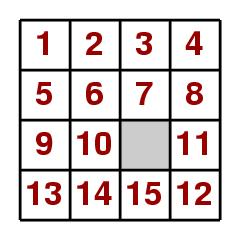By sliding the 12 down one permutation is performed (12 *) which is odd. To get back to the home state therefore the solution will be an odd permutation as the empty space was left on a white square. Similarly if the starting state is (12 * 11) from the home state, one can tell that the solution is possible with an even permutation. In the same way one can look at any shuffled starting position: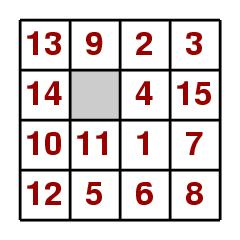Here the permutations are
(1 11 10 9 2 3 4 7 12 13 8 * 6 15)(5 14)
which is a combination of two odd permutations so is even. The empty square is found on a green position so one can say that this problem is solvable.

### The 14-15 Puzzle and other problems

Now Loyd's 14-15 puzzle shall be examined: this is the starting state which looks like this:
Even though this appears to be so close to the final solution, one can tell from the cycle that it is not solvable, because (14 15) is an odd permutation and the space on a green position, ie odd parity.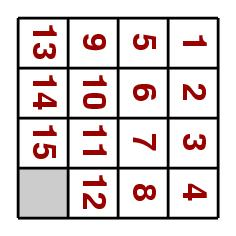Other starting states that can be looked at are the effect of rotating the grid clockwise 90 degrees. The permutation is
(1 4 * 13)(2 8 15 9)(3 12 14 5)(6 7 11 10)
which is a combination of 4 odd permutations which is itself even. The empty space is on a white position so this problem (including the problem of having the tiles on their sides) is unsolvable.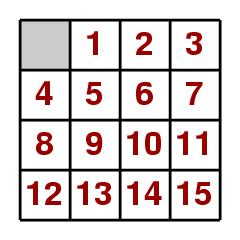If one takes the problem of having the empty space at the beginning rather than at the end, the permutation cycle is
(1 2 3 4 5 6 7 8 9 10 11 12 13 14 15 *)
which is an odd permutation with the space on a green position so it is an unsolvable problem.

### Finding Solutions-Simple

Of course after working out whether a particular problem is solvable, it would then be useful to be able to find a solution. One logical process to follow is to get the tiles in place in order, ie by getting the 1 into place, then the 2,3,4 etc.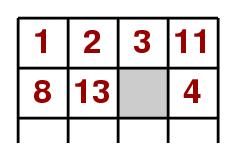However one may quickly find that by placing the 3 in place, the 4 can not fit without disturbing the 3 again. From the work before, it is known that a permutation of (11 4) on the top right-hand 2 by 2 grid is not legal by sliding so instead permutations of a 2 by 3 grid are used.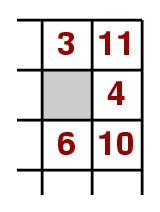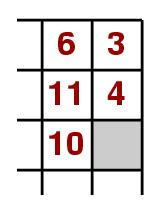This is the same as a permutation of (4 10 6 *)(6 3 11 *)(11 * 4)(3 6 11 10 * 4)= (11 6 * 4). In a similar way, the 7 and 8 can be placed in the correct positions. The bottom two lines will have similar problems; the 9 and 13 need to be in the right order then cycled round to their positions, and finally one is left with another 3 by 2 grid with the 10 and 14 to be placed first like the 3 and 4, then the last three rotated to get home! Of course it makes sense to think about the other numbers and where they should eventually end up, and so move them in those particular directions.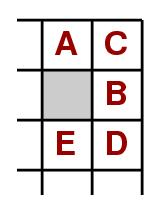For the general case if a tile, B needs to get into a upper right corner (either of the grid, or previously positioned tiles) in the order A B at the top, the cycle of moves from this position is thus:
(A C B D E *)(D B C E *)(B A E C*) in terms of the legal slides.
These motions can then be used for any part of the problem in a corner, just mirror the permutation to fit the corner.

### Finding Solutions-Fastest

Obviously trying to solve the problem like this is not the fastest (ie the one with the least number of moves) as one tends to move some tiles in the wrong direction unnecessarily as the first ones are concentrated on individually. As one can see in arranging the corner piece, it is sometimes necessary to go backwards to be able to go forwards, but these motions ought to be limited where possible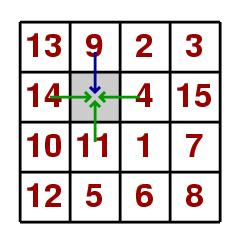A computer could be used to evaluate all possible solutions and then show the quickest one. It would look at all possible moves at each node- where there is a branching off of possible solutions. For example in the previous problem (shown again to the left), the starting position has a node of different 4 paths or branches; the 4, 9, 11 or 14 could be moved. If the 9 is chosen to be moved, the new node would have 3 branches, but the 9 would be discounted as it leads back to the original position.
One way of getting the computer to perform the search is to make it evaluate possibilities at each node, to pick one path and follow it until either the home state is reached or a dead end. If a dead end is reached, the computer goes back to the last node and takes a different path. However, one can not be sure that this is the fastest, as it discards all other possibilities once it has found a solution. Instead the computer could examine all nodes that are one permutation from the starting position. If none of these are the solution they are expanded to nodes 2 single permutations away from the starting state, etc until the home state is found, so this will be the shortest. This however is very complicated and certainly not something a human could do easily. There are many sites on the internet that have programs to find the quickest solution (see sources) so instead the problem of whether a person can solve the fifteen puzzle in the shortest solution will be examined.One thing a human can do is to work out an heuristic or best fit- an estimate of the number of moves a tile would take to get from a particular position on the grid to the home state. The heuristic really wants to be either optimistic or correct as it will show the minimum number of moves expected to get home. It is worked out by looking at the position of each tile in the starting state and calculating the number of moves it would take to get to its home place if the space were adjacent to it, in the direction of home. In the example, the 1 would need 4 moves to get home (so the heuristic is 4), the 13 would need 3, the 3, 1 move. It is known that the heuristic calculated in this way is optimistic or accurate, because if the empty space were to the left of its position now, the 13 could get to its home place in the heuristic's estimated number of moves. However as it is not, the heuristic would be larger as the tile can not be simply lifted up. The heuristic of a particular problem is just the sum of all the individual heuristics.

It would seem sensible to work out the total heuristic for a particular problem and then follow the path of best fit where the value of the heuristic decreases. For the example taken, the heuristic at the start would be 4 + 1 + 1 +...+ 3 + 3 + 3 = 36 for the tiles in chronological order. To decrease the heuristic, the 9 or 14 could be moved, as they are the only two moves that move the tile closer to its home position. Moving one tile in its home direction decreases the value of the heuristic by one. A person can keep track of the moves made and where the corresponding best fit nodes are. When a dead end is reached, the person should go back to the last node and examine the other branches, until a solution is found. However in practice this does not work out. It was seen from the process of moving corner pieces into position that it is necessary to move tiles in what seems the wrong direction in order to solve the problem.

In conclusion, a human can combine both ways of solving the problem; through simple logical solving and by trying to move the tiles in the direction of best fit. In this way a solution will be found that will be reasonably short, and probably shorter than any solution the person would have found by trial and error. Obviously it does not take into account the time needed to work out the heuristic at the start. Hopefully, understanding the principles used in the fifteen puzzle would help a person understand the problem and to find the best way to go about achieving a solution, whether they want a solution that is fast in time or number of moves, or just to solve it for fun.

### Sources:

W. W. Rouse Ball, Mathematical Recreations and Essays, Chapter XI Miscellaneous problems, 10th ED. Macmillan and Co. 1937.
John Ewing and Czes Kosniowski, Puzzle It Out - Cubes, Groups and Puzzles, Cambridge University Press 1982.
www.geocities.com/dariuscooper/puzzle/
www.geocities.com/jaapsch/puzzles/fifteen.htm
mitglied.lycos.de/jkoeller/15puzzle.htm

MATH 308 Euclidean Geometry Project, 2002
Hazel Grant